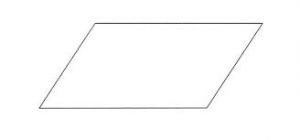# Program to find the Area of a Parallelogram

Given the sides of a Parallelogram, task is calculate the area of a Parallelogram.Examples:

```Input: base = 30, height = 40
Output: 1200.000000
As Area of parallelogram = base * height,
Therefore, Area = 30 * 40 = 1200.00
```

Approach:

Area of parallelogram = base * height

Below is the implementation of the above approach:

## C++

 `#include ` `using` `namespace` `std; ` ` `  `// function to calculate the area ` `float` `CalArea(``float` `base, ``float` `height) ` `{ ` `    ``return` `(base * height); ` `} ` `// driver code ` `int` `main() ` `{ ` `    ``float` `base, height, Area; ` `    ``base = 30; ` `    ``height = 40; ` ` `  `    ``// function calling ` `    ``Area = CalArea(base, height); ` `    ``// displaying the area ` `    ``cout << ``"Area of Parallelogram is :"` `<< Area; ` `    ``return` `0; ` `} `

## C

 `#include ` ` `  `// function to calculate the area ` `float` `CalArea(``float` `base, ``float` `height) ` `{ ` `    ``return` `(base * height); ` `} ` ` `  `// driver code ` `int` `main() ` `{ ` `    ``float` `base, height, Area; ` `    ``base = 30; ` `    ``height = 40; ` ` `  `    ``// function calling ` `    ``Area = CalArea(base, height); ` ` `  `    ``// displaying the area ` `    ``printf``(``"Area of Parallelogram is : %f\n"``, Area); ` `    ``return` `0; ` `} `

## Java

 `public` `class` `parallelogram { ` `    ``public` `static` `void` `main(String args[]) ` `    ``{ ` `        ``int` `base = ``30``; ` `        ``int` `height = ``40``; ` ` `  `        ``// formula for calculating the area ` `        ``int` `area_parallelogram = base * height; ` ` `  `        ``// displayin g the area ` `        ``System.out.println(``"Area of the parallelogram = "` `+ area_parallelogram); ` `    ``} ` `} `

## Python

 `base ``=` `30`  `height ``=` `40`   ` `  `# formula for finding the area ` `area_parallelogram ``=` `base ``*` `height ` ` `  `# displaying the output ` `print``(``"Area of the parallelogram = "``+``str``(area_parallelogram)) `

## C#

 `using` `System; ` `class` `parallelogram  ` `{ ` `public` `static` `void` `Main() ` `{ ` `    ``int` `b_ase = 30; ` `    ``int` `height = 40; ` ` `  `    ``// formula for calculating the area ` `    ``int` `area_parallelogram = b_ase * height; ` ` `  `    ``// displayin g the area ` `    ``Console.WriteLine(``"Area of the parallelogram = "` `+  ` `                                  ``area_parallelogram); ` `} ` `} ` ` `  `// This code is contributed by vt_m `

## PHP

 `  `

Output:

```Area of Parallelogram is : 1200.000000
```

My Personal Notes arrow_drop_upCheck out this Author's contributed articles.

If you like GeeksforGeeks and would like to contribute, you can also write an article using contribute.geeksforgeeks.org or mail your article to contribute@geeksforgeeks.org. See your article appearing on the GeeksforGeeks main page and help other Geeks.

Please Improve this article if you find anything incorrect by clicking on the "Improve Article" button below.

Improved By : vt_m